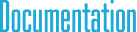#cyIVector.h File Reference

## Detailed Description

2D, 3D, 4D, and ND integer vector classes.

`#include "cyCore.h"`

## Classes

class  IVec< T, N >

class  IVec2< T >

class  IVec3< T >

class  IVec4< T >

## Typedefs

typedef IVec2< int8_t > IVec2b

typedef IVec3< int8_t > IVec3b

typedef IVec4< int8_t > IVec4b

typedef IVec2< uint8_t > IVec2ub

typedef IVec3< uint8_t > IVec3ub

typedef IVec4< uint8_t > IVec4ub

typedef IVec2< int16_t > IVec2s

typedef IVec3< int16_t > IVec3s

typedef IVec4< int16_t > IVec4s

typedef IVec2< uint16_t > IVec2us

typedef IVec3< uint16_t > IVec3us

typedef IVec4< uint16_t > IVec4us

typedef IVec2< int32_t > IVec2i

typedef IVec3< int32_t > IVec3i

typedef IVec4< int32_t > IVec4i

typedef IVec2< uint32_t > IVec2ui

typedef IVec3< uint32_t > IVec3ui

typedef IVec4< uint32_t > IVec4ui

typedef IVec2< int64_t > IVec2l

typedef IVec3< int64_t > IVec3l

typedef IVec4< int64_t > IVec4l

typedef IVec2< uint64_t > IVec2ul

typedef IVec3< uint64_t > IVec3ul

typedef IVec4< uint64_t > IVec4ul

typedef cy::IVec2b cyIVec2b

typedef cy::IVec3b cyIVec3b

typedef cy::IVec4b cyIVec4b

typedef cy::IVec2ub cyIVec2ub

typedef cy::IVec3ub cyIVec3ub

typedef cy::IVec4ub cyIVec4ub

typedef cy::IVec2s cyIVec2s

typedef cy::IVec3s cyIVec3s

typedef cy::IVec4s cyIVec4s

typedef cy::IVec2us cyIVec2us

typedef cy::IVec3us cyIVec3us

typedef cy::IVec4us cyIVec4us

typedef cy::IVec2i cyIVec2i

typedef cy::IVec3i cyIVec3i

typedef cy::IVec4i cyIVec4i

typedef cy::IVec2ui cyIVec2ui

typedef cy::IVec3ui cyIVec3ui

typedef cy::IVec4ui cyIVec4ui

typedef cy::IVec2l cyIVec2l

typedef cy::IVec3l cyIVec3l

typedef cy::IVec4l cyIVec4l

typedef cy::IVec2ul cyIVec2ul

typedef cy::IVec3ul cyIVec3ul

typedef cy::IVec4ul cyIVec4ul

## § IVec2b

 typedef IVec2< int8_t> IVec2b

8-bit signed byte (int8_t) 2D integer vector class

## § IVec3b

 typedef IVec3< int8_t> IVec3b

8-bit signed byte (int8_t) 3D integer vector class

## § IVec4b

 typedef IVec4< int8_t> IVec4b

8-bit signed byte (int8_t) 4D integer vector class

## § IVec2ub

 typedef IVec2 IVec2ub

8-bit unsigned byte (uint8_t) 2D integer vector class

## § IVec3ub

 typedef IVec3 IVec3ub

8-bit unsigned byte (uint8_t) 3D integer vector class

## § IVec4ub

 typedef IVec4 IVec4ub

8-bit unsigned byte (uint8_t) 4D integer vector class

## § IVec2s

 typedef IVec2< int16_t> IVec2s

16-bit signed short (int16_t) 2D integer vector class

## § IVec3s

 typedef IVec3< int16_t> IVec3s

16-bit signed short (int16_t) 3D integer vector class

## § IVec4s

 typedef IVec4< int16_t> IVec4s

16-bit signed short (int16_t) 4D integer vector class

## § IVec2us

 typedef IVec2 IVec2us

16-bit unsigned short (uint16_t) 2D integer vector class

## § IVec3us

 typedef IVec3 IVec3us

16-bit unsigned short (uint16_t) 3D integer vector class

## § IVec4us

 typedef IVec4 IVec4us

16-bit unsigned short (uint16_t) 4D integer vector class

## § IVec2i

 typedef IVec2< int32_t> IVec2i

32-bit signed integer (int32_t) 2D integer vector class

## § IVec3i

 typedef IVec3< int32_t> IVec3i

32-bit signed integer (int32_t) 3D integer vector class

## § IVec4i

 typedef IVec4< int32_t> IVec4i

32-bit signed integer (int32_t) 4D integer vector class

## § IVec2ui

 typedef IVec2 IVec2ui

32-bit unsigned integer (uint32_t) 2D integer vector class

## § IVec3ui

 typedef IVec3 IVec3ui

32-bit unsigned integer (uint32_t) 3D integer vector class

## § IVec4ui

 typedef IVec4 IVec4ui

32-bit unsigned integer (uint32_t) 4D integer vector class

## § IVec2l

 typedef IVec2< int64_t> IVec2l

64-bit signed long (int64_t) 2D integer vector class

## § IVec3l

 typedef IVec3< int64_t> IVec3l

64-bit signed long (int64_t) 3D integer vector class

## § IVec4l

 typedef IVec4< int64_t> IVec4l

64-bit signed long (int64_t) 4D integer vector class

## § IVec2ul

 typedef IVec2 IVec2ul

64-bit unsigned long (uint64_t) 2D integer vector class

## § IVec3ul

 typedef IVec3 IVec3ul

64-bit unsigned long (uint64_t) 3D integer vector class

## § IVec4ul

 typedef IVec4 IVec4ul

64-bit unsigned long (uint64_t) 4D integer vector class

## § cyIVec2b

 typedef cy::IVec2b cyIVec2b

8-bit signed integer (int8_t) 2D integer vector class

## § cyIVec3b

 typedef cy::IVec3b cyIVec3b

8-bit signed integer (int8_t) 3D integer vector class

## § cyIVec4b

 typedef cy::IVec4b cyIVec4b

8-bit signed integer (int8_t) 4D integer vector class

## § cyIVec2ub

 typedef cy::IVec2ub cyIVec2ub

8-bit unsigned integer (uint8_t) 2D integer vector class

## § cyIVec3ub

 typedef cy::IVec3ub cyIVec3ub

8-bit unsigned integer (uint8_t) 3D integer vector class

## § cyIVec4ub

 typedef cy::IVec4ub cyIVec4ub

8-bit unsigned integer (uint8_t) 4D integer vector class

## § cyIVec2s

 typedef cy::IVec2s cyIVec2s

16-bit signed integer (int16_t) 2D integer vector class

## § cyIVec3s

 typedef cy::IVec3s cyIVec3s

16-bit signed integer (int16_t) 3D integer vector class

## § cyIVec4s

 typedef cy::IVec4s cyIVec4s

16-bit signed integer (int16_t) 4D integer vector class

## § cyIVec2us

 typedef cy::IVec2us cyIVec2us

16-bit unsigned integer (uint16_t) 2D integer vector class

## § cyIVec3us

 typedef cy::IVec3us cyIVec3us

16-bit unsigned integer (uint16_t) 3D integer vector class

## § cyIVec4us

 typedef cy::IVec4us cyIVec4us

16-bit unsigned integer (uint16_t) 4D integer vector class

## § cyIVec2i

 typedef cy::IVec2i cyIVec2i

32-bit signed integer (int32_t) 2D integer vector class

## § cyIVec3i

 typedef cy::IVec3i cyIVec3i

32-bit signed integer (int32_t) 3D integer vector class

## § cyIVec4i

 typedef cy::IVec4i cyIVec4i

32-bit signed integer (int32_t) 4D integer vector class

## § cyIVec2ui

 typedef cy::IVec2ui cyIVec2ui

32-bit unsigned integer (uint32_t) 2D integer vector class

## § cyIVec3ui

 typedef cy::IVec3ui cyIVec3ui

32-bit unsigned integer (uint32_t) 3D integer vector class

## § cyIVec4ui

 typedef cy::IVec4ui cyIVec4ui

32-bit unsigned integer (uint32_t) 4D integer vector class

## § cyIVec2l

 typedef cy::IVec2l cyIVec2l

64-bit signed integer (int64_t) 2D integer vector class

## § cyIVec3l

 typedef cy::IVec3l cyIVec3l

64-bit signed integer (int64_t) 3D integer vector class

## § cyIVec4l

 typedef cy::IVec4l cyIVec4l

64-bit signed integer (int64_t) 4D integer vector class

## § cyIVec2ul

 typedef cy::IVec2ul cyIVec2ul

64-bit unsigned integer (uint64_t) 2D integer vector class

## § cyIVec3ul

 typedef cy::IVec3ul cyIVec3ul

64-bit unsigned integer (uint64_t) 3D integer vector class

## § cyIVec4ul

 typedef cy::IVec4ul cyIVec4ul

64-bit unsigned integer (uint64_t) 4D integer vector class